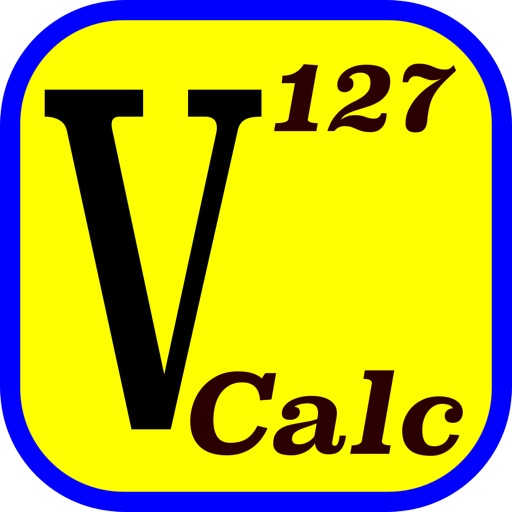## V-Calc Lite is a textbook-display programmable scientific calculator for iPhone that is designed to solve real problems in engineering and science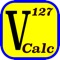# V-Calc Lite

by Staghorn Ridge Software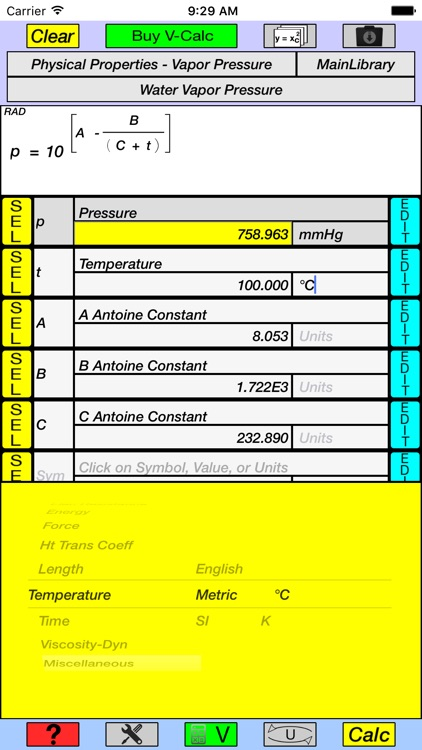V-Calc Lite is a textbook-display programmable scientific calculator for iPhone that is designed to solve real problems in engineering and science.### App Details

Version
1.0
Rating
NA
Size
7Mb
Genre
Utilities Productivity
Last updated
April 1, 2016
Release date
April 1, 2016

### App Screenshots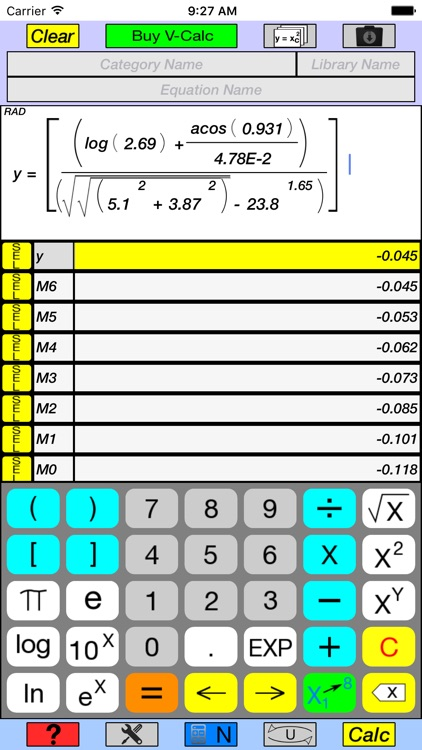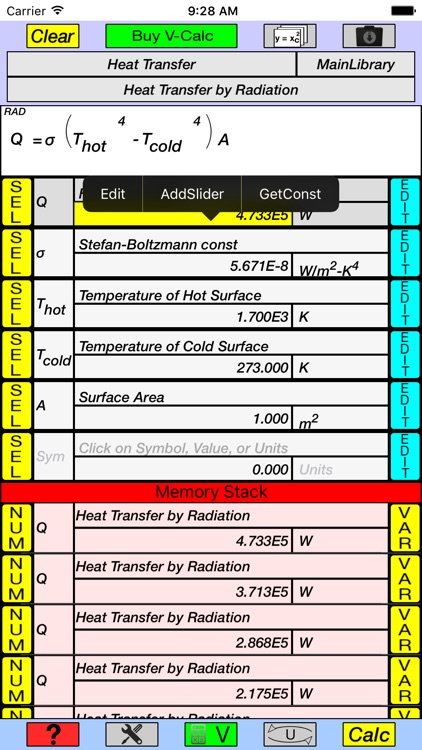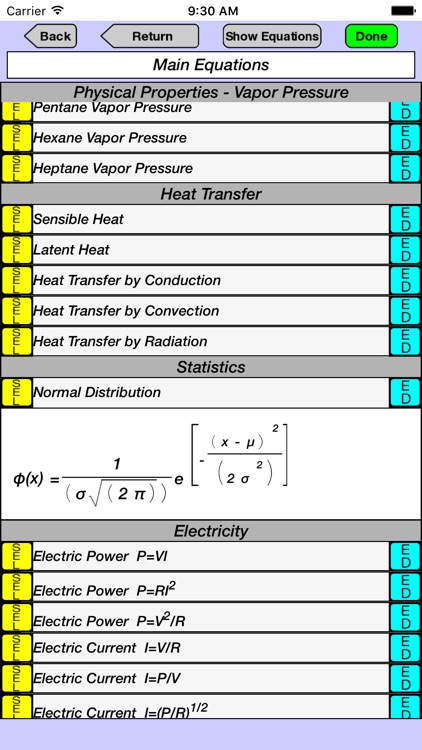### App Store Description

V-Calc Lite is a textbook-display programmable scientific calculator for iPhone that is designed to solve real problems in engineering and science.

• Equations are easily created and displayed in their natural form, as they would appear in a textbook.
• Well-organized Main, Trigonometric, and English keypads. (the full version of V-Calc also has a Greek keypad, Hyperbolic functions, and the Factorial function)
• Variables, units, and constants can be created and used in equations, with no limit on the number of each of these. (the full version allows user-created units, constants, and explicit equations to be saved to libraries under user-defined categories.)
• 10 Categories of Units. (the full version has 59 Categories)
• Can be used as a stand-alone Unit Converter, or to convert the results from the Scientific Calculator.
• Units can also be converted for use as input to the Scientific Calculator.
• Units can be quickly retrieved, to convert directly (Unit Conversion Mode), or for insertion into the variable table, by use of picker dials to select categories (area, mass, volume), systems (english, metric, SI) and units (kg/s, °C, m/h)..
• Unit conversions are made simultaneously, and are displayed as a table of all other units of the same category.
• Full subscripting and superscripting for variable and constant symbols, and units.
• Can use period or comma as decimal separator.
• Multiplication operator is optional in most cases.
• Numbers use decimal number precision and can have a mantissa of up to 38 digits with a scientific notation range of 10^-128 to 10^127.
• Exponent, multiplication, division, addition, and subtraction operations maintain decimal number precision. All other operations and functions are executed with long double precision.
• Complete English keypad, with small and large caps, for creating variable and constant symbols and units.
• Trigonometric keypad with inverse functions.
• Keypads appear automatically, depending on current need.
• Decimal or scientific notation with 0 to 37 decimal places.
• Degrees or radians for trigonometric functions.
• Includes sample equation and constant libraries.
• Equation library has a summary page for each equation, which includes a display of the equation, its variables in table format, and a detailed description.
• Number Mode allows rapid entry of numbers, operators and functions to create and execute an equation in textbook-display format.
• Variable Mode allows the creation of a variable table with symbols, descriptions, units, and values, which are then readily entered along with numbers, operators, and functions to create and execute an equation in textbook-display format. Alternatively, equations can be retrieved from one of the categories in the equation library. The result of the calculation is automatically converted to all units in the same category and displayed in a table.
• Unit Conversion Mode allows unit conversions to be made directly by entering a number and its units. The result is displayed in a table as described for Variable Mode.
• Button selector allows variable symbols or their numeric values to be alternately displayed in equations.
• Memory stack maintains values, variable symbols, and equation names of several hundred previous calculations, which can be easily accessed for future calculations.
• Previously used equations are maintained in memory and can be re-accessed by means of a drop-down selection window.
• Help screen overlay provides pop-up description of the function of key buttons.
• Tutorials demonstrate the calculator’s capabilities by programmatically stepping through the creation and execution of several different equations.
• E-mail contact is accessed through a button provided in the Help/Tutorial screens.
• (Idle timer for full version is disabled so that screen does not dim and calculator is available continuously until deselected by user)
• Works with iPhone 4s through iPhone 6s, 6s Plus, and iPad.